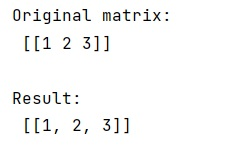# Python - How to Convert a NumPy Matrix to List?

Learn, how to convert a NumPy matrix to list in Python?
Submitted by Pranit Sharma, on March 25, 2023

## Making a List from NumPy Matrix

A list is a collection of heterogeneous elements and it is mutable in nature. Elements inside the list are encapsulated in square brackets. A list is considered a series or collection and we can perform operations like insert, update, and delete with its elements.

Suppose that we are given a numpy matrix and we need to convert it into a flat Python list.

To convert a NumPy matrix to list, we can simply use .tolist() function on the numpy matrix which will directly convert the matrix into a flat 1D list.

Let us understand with the help of an example,

## Python code to convert a NumPy matrix to list

```# Import numpy
import numpy as np

# Creating a numpy matrix
mat = np.matrix([1, 2, 3])

# Display original matrix
print("Original matrix:\n",mat,"\n")

# Converting matrix into a list
res = mat.tolist()

# Display result
print("Result:\n",res)
```

### Output